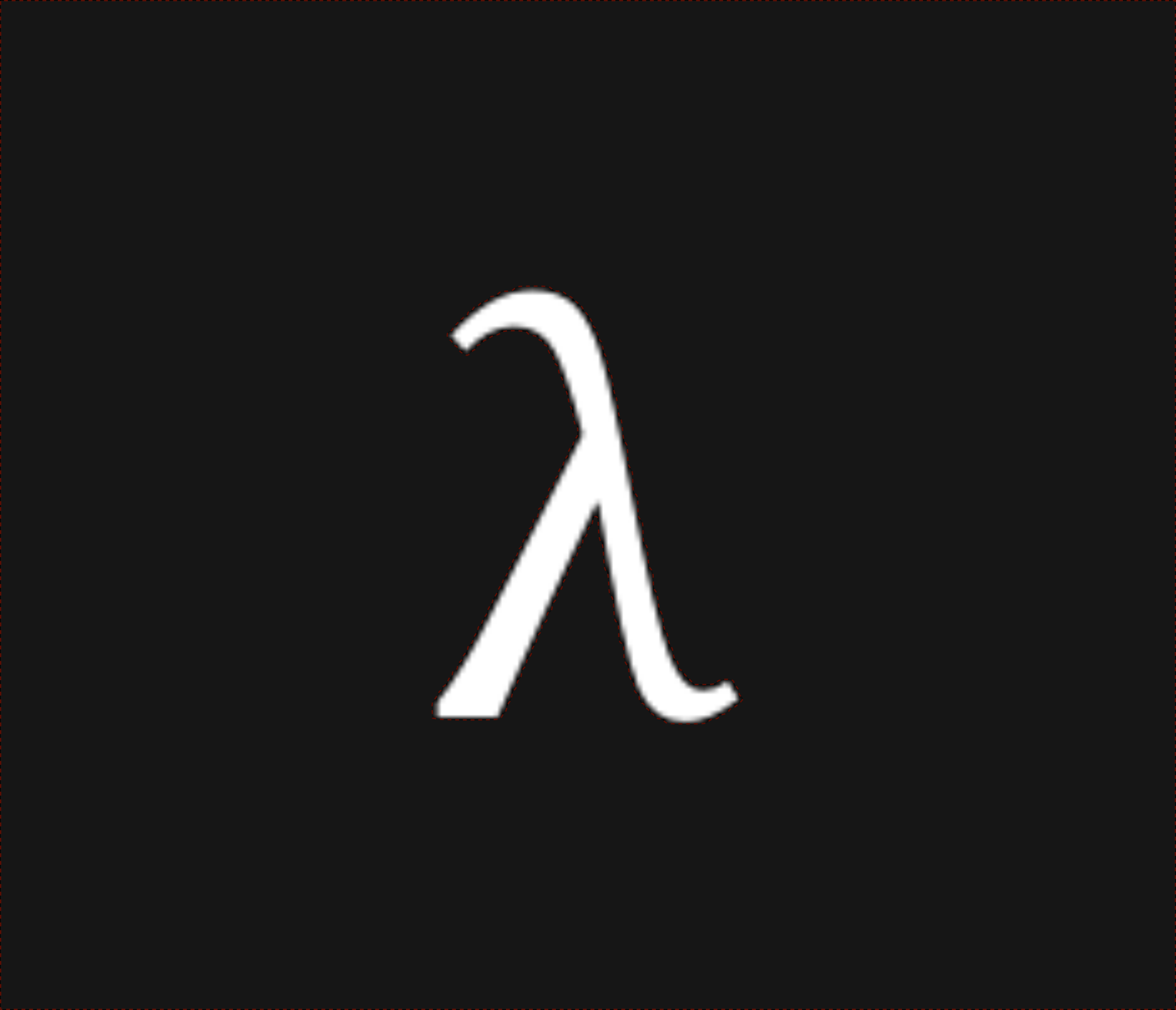nixpulvis

## Euler's Identity 631 words written by Nathan Lilienthal

$e^{\pi i} + 1 = 0$

This equation is supremely beautiful and bewildering. It uses 5 very fundamental objects: $$0$$, $$1$$, $$\pi = 3.141\ldots$$, $$e = 2.718\ldots$$, and $$i = \sqrt{-1}$$, along with 4 operations: $$a + b$$ (addition), $$a b$$ (multiplication), $$a^b$$ (exponentiation), and finally $$a = b$$ (equality, identity).

$e^{\frac{\pi i}{2}} = i \\ e^{\pi i} = (e^{\frac{\pi i}{2}})^2 = i^2 = -1 \\ e^{\frac{3\pi i}{2}} = (e^{\frac{\pi i}{2}})^3 = i^3 = -i \\ e^{2\pi i} = (e^{\frac{\pi i}{2}})^4 = i^4 = 1$

Then it repeats…

$e^{\frac{5\pi i}{2}} = (e^{\frac{\pi i}{2}})^5 = i^5 = i \\ \vdots$

If you can’t see the “whole” picture yet (can anyone?), this might help. Our cyclic circular sidekicks, $$\sin$$ and $$\cos$$ are here to enlighten us.

Euler’s identity is a special case of Euler’s formula, which states:

$e^{i \theta} = \cos(\theta) + i \sin(\theta)$

Substitute $$\pi$$ (or in general, $$n\pi$$) for $$\theta$$ and we get back our identity.

\begin{align} \cos(\pi) + i \sin(\pi) = -1 + 0 =\ & e^{\pi i} = i^2 \\ & e^{\pi i} + 1 = 0 \end{align}
Tangent on Fourier Series

TODO: This information is only a sketch, and as such is somewhat sketchy.

Plot[
sin(theta)/pi,
(3/2) sin(theta)/pi,
2     sin(theta)/pi,
(5/2) sin(theta)/pi
]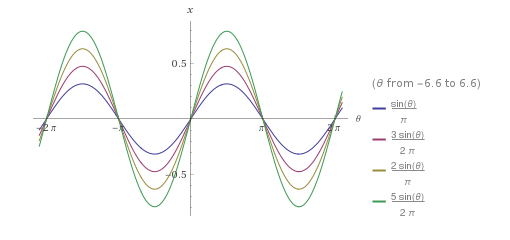Unlike the “Euler series” we above, which is purely constructive, a square wave can be constructed with the Fourier series:

$\sin(2 \pi \theta), \frac{1}{3} \sin(6 \pi \theta), \frac{1}{5} \sin(10 \pi \theta), ...$
Plot[
sin(2 pi theta),
(1/3) sin(6 pi theta),
(1/5) sin(10 pi theta)
]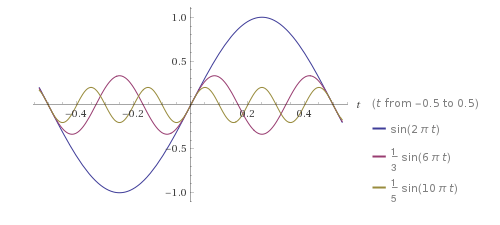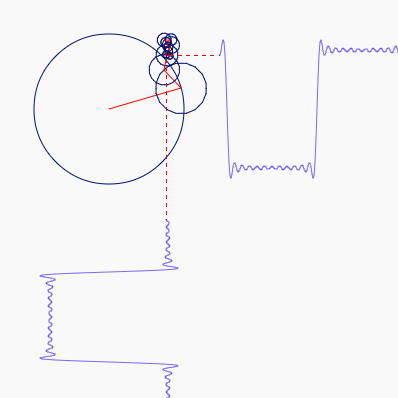And a saw wave can be constructed by another:

$\sin(\pi \theta), \sin(\frac{\pi \theta}{2}), \sin(\frac{\pi \theta}{4}), \ldots$
Plot[
sin(pi theta),
sin((pi theta) / 2),
sin((pi theta) / 4)
]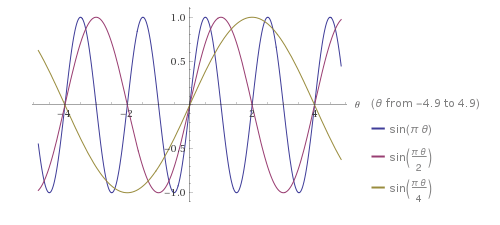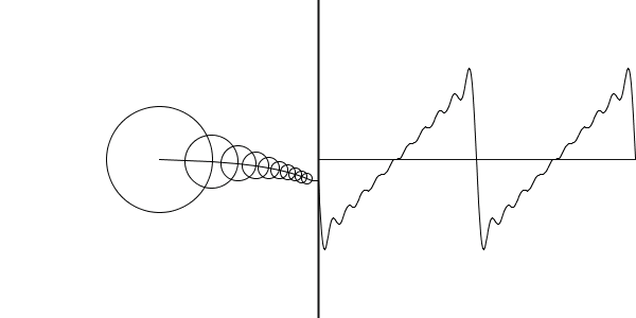Euler’s identity can be geometrically interpreted as saying that rotating any point $$\pi$$ radians around an origin of a complex plane has the same effect as reflecting the point across the origin.

Fundamentally, Euler’s Identity is a root of unity, meaning that it is a solution for $$z$$ in the equation $$z^n = 1$$.

$z = e^{2\pi i} = i^4$

This is the definition for a more general identity which states that the $$n$$th roots of unity, for $$n \gt 1$$, add up to $$0$$.

$\sum_{k=0}^{n-1} e^{2\pi i\frac{k}{n}} = 0$

Euler’s identity is the $$n = 2$$ case of this equation.

$e^0 + e^{\pi i} = e^{\pi i} + 1 = 0$
A fun fact from a friend.

My friend Ryan showed me an interesting approximation of $$e$$ which uses numbers $$1, 2, 3, 4, 5, 6, 7, 8, 9$$ each once:

$e \approx \left(1 + 9^{-4^{6 \times 7}}\right)^{3^{2^{85}}}$

This may be less surprising if we recall that $$e$$ can be defined as:

$e = \lim_{n \to \infty} \left(1 + \frac{1}{n}\right)^n$

So we have $$n \gets 3^{2^{85}} \approx \left(9^{-4^{6 \times 7}}\right)^{-1}$$, which substituting in the above, gives an approximation of $$e$$.

Wanna compute e in Brainfuck? $$\hphantom{nothing} \\ e = 2.718281828459\ldots$$

git clone https://github.com/nixpulvis/brainfuck
cd brainfuck
cargo run fixtures/e.bf


>>>>++>+>++>+>>++<+[
[>[>>[>>>>]<<<<[[>>>>+<<<<-]<<<<]>>>>>>]+<]>-
>>--[+[+++<<<<--]++>>>>--]+[>>>>]<<<<[<<+<+<]<<[
>>>>>>[[<<<<+>>>>-]>>>>]<<<<<<<<[<<<<]
>>-[<<+>>-]+<<[->>>>[-[+>>>>-]-<<-[>>>>-]++>>+[-<<<<+]+>>>>]<<<<[<<<<]]
>[-[<+>-]]+<[->>>>[-[+>>>>-]-<<<-[>>>>-]++>>>+[-<<<<+]+>>>>]<<<<[<<<<]]<<
]>>>+[>>>>]-[+<<<<--]++[<<<<]>>>+[
>-[
>>[--[++>>+>>--]-<[-[-[+++<<<<-]+>>>>-]]++>+[-<<<<+]++>>+>>]
<<[>[<-<<<]+<]>->>>
]+>[>>>>]-[+<<<<--]++<[
[>>>>]<<<<[
-[->--[<->+]++<[[>-<+]++[<<<<]+>>+>>-]++<<<<-]
>-[+[<+[<<<<]>]<+>]+<[->->>>[-]]+<<<<
]
]>[<<<<]>[
-[
-[
+++++[>++++++++<-]>-.>>>-[<<<----.<]<[<<]>>[-]>->>+[
[>>>>]+[-[->>>>+>>>>>>>>-[-[+++<<<<[-]]+>>>>-]++[<<<<]]+<<<<]>>>
]+<+<<
]>[
-[
->[--[++>>>>--]->[-[-[+++<<<<-]+>>>>-]]++<+[-<<<<+]++>>>>]
<<<<[>[<<<<]+<]>->>
]<
]>>>>[--[++>>>>--]-<--[+++>>>>--]+>+[-<<<<+]++>>>>]<<<<<[<<<<]<
]>[>+<<++<]<
]>[+>[--[++>>>>--]->--[+++>>>>--]+<+[-<<<<+]++>>>>]<<<[<<<<]]>>
]>
]

This program computes the transcendental number e, in decimal. Because this is
infinitely long, this program doesn't terminate on its own; you will have to
kill it. The fact that it doesn't output any linefeeds may also give certain
implementations trouble, including some of mine.

(c) 2016 Daniel B. Cristofani
http://brainfuck.org/